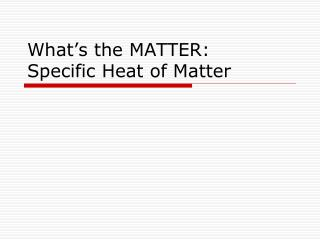DownloadDownload PresentationWhat’s the MATTER: Specific Heat of Matter

# What’s the MATTER: Specific Heat of Matter

Download Presentation## What’s the MATTER: Specific Heat of Matter

- - - - - - - - - - - - - - - - - - - - - - - - - - - E N D - - - - - - - - - - - - - - - - - - - - - - - - - - -
##### Presentation Transcript

1. What’s the MATTER:Specific Heat of Matter

2. Matter, Specific Heat of Matter At the conclusion of our time together, you should be able to: Define specific heat Use specific heat to determine energy changes

3. Thermochemistry • Some Definitions: • Calorimeter – a device to measure the energy absorbed or released as heat in a chemical or physical change • Temperature – measure of the average kinetic energy of the particles in a sample of matter • Joule – the SI unit of heat

4. Thermochemistry • Some Definitions: • Heat – energy transferred between samples of matter • Specific Heat – the amount of energy required to raise the temperature of one gram of a substance by one Celsius degree or one Kelvin • 1 Calorie/4.184 Joules – will do the above with water

5. Thermochemistry • Some Definitions: • Enthalpy (Heat) of Fusion – amount of energy gained or lost by a system as heat during melting or freezing • Enthalpy (Heat) of Vaporization – amount of energy gained or lost by a system as heat during boiling or condensation

6. Specific Heat Calculations: • q = cp x m x t: • q = energy lost or gained • cp = specific heat of the substance at a specific pressure • m = mass of the sample • t = change in temperature (final – initial)

7. Practice #1 • q = cp x m x t: • q = 59.912 J • cp = x • m = 36.359 g • t = 152.0 oC 59.912 J = (x)(36.359 g)(152.0 oC) = 0.01084 J/goC

8. Practice #2 • q = cp x m x t: • q = -800. J • cp= 0.4210 J/g oC • m = 73.174 g • t = (x – 102.0 oC) -800. J = 0.4210 J/goC (73.174 g)(x – 102.0 oC) -800. = 30.81x – 3142 2342 = 30.81x = 76.0 oC

9. Matter, Specific Heat of Matter Let’s see if you can : Define specific heat Use specific heat to determine energy changes

10. Define Specific Heat • Specific Heat – the amount of energy required to raise the temperature of one gram of a substance by one Celsius degree or one Kelvin

11. Practice #3 • q = cp x m x t: • q = -185.4 J • cp= 0.440 J/g oC • m = x g • t = -1475 oC -185.4 J = (0.440 J/goC )(x)(-1475 oC) -185.4 J = -649 Jg = 0.29 g

12. Practice #4 • q = cp x m x t: • q = x J • cp= 0.0335 cal/goC (4.184 J/cal) • m = 152.00 g • t = -51.5oC x = (0.140164 J/goC )(152.00 g)(-51.5 oC) = -1.10 x 103 J